The Fourier series of a rectangle function

Today, we're going to play with a couple of sine functions. We're interested in functions of the form $f_n(x) = sin(2\pi \, n \, x)$. Let's plot that function on the interval $[0; 1]$.

In :
%pylab inline
Populating the interactive namespace from numpy and matplotlib
In :
x = arange(0, 1, 0.01)
sin_n = lambda n: sin(2 * pi * n * x)
In :
plot(x, sin_n(1))
Out:
[]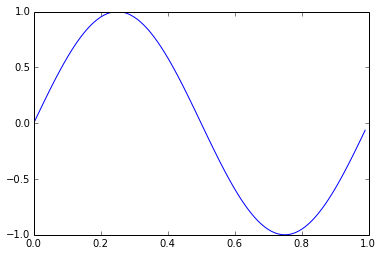What happens if we display successive $f_n$ on this interval?

In :
for i in [1, 2, 5, 8, 9, 12]:
plot(x, sin_n(i), label='n = %i' % i)
legend()
Out: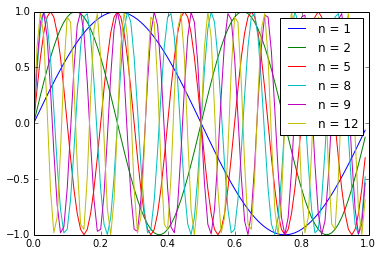But what happens if we sum them?

In :
sum_i_j = lambda i,j: sum([sin_n(k) for k in range(i, j+1)], axis=0)
In :
for i in [1, 2, 3, 4, 5, 10, 20, 40]:
plot(x, sum_i_j(1, i))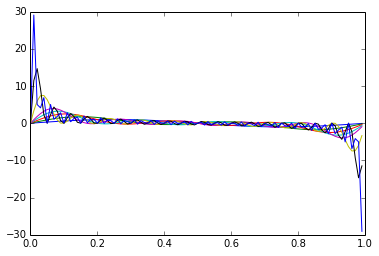What does it look like? It looks like all the high frequencies are cancelling each other out to leave just a sort of impulse function.

It turns out that the property I was looking form is linked to the Fourier series of a square waveform. Reference can be found here.

If a square waveform of period T is defined by $$\left\{ \begin{array}{l l} f(t)= 1 \text{ if } t= T/2 \end{array} \right.$$

In :
plot(x, x<1/2.)
ylim(-1.1, 2.1)
Out:
(-1.1, 2.1)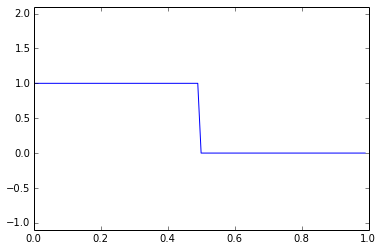This function, taken as periodic, can be approximated by a Fourier series with formula: $$F(t) = 1/2 + \sum_{p=0}^{\infty}\frac{2}{\pi (2p+1)} \sin(2 \pi (2 p + 1) t)$$

In :
F_n = lambda n: (1/2. + sum([2./pi/(2*i+1) * sin(2 * pi * (2*i+1) * x) for i in range(n+1)], axis=0))
In :
plot(x, x<1/2.)
for i in [0, 1, 2, 3, 5, 10]:
plot(x, F_n(i))
ylim(-1.1, 2.1)
Out:
(-1.1, 2.1)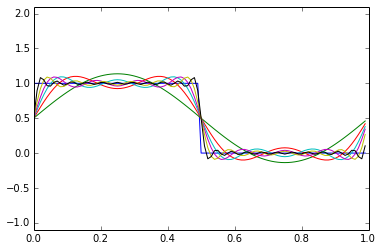With a high number of harmonics, we get the following approximation:

In :
n = 20
plot(x, x<1/2.)
plot(x, F_n(n))
ylim(-1.1, 2.1)
title('square wave approximation with %i terms' % n)
Out: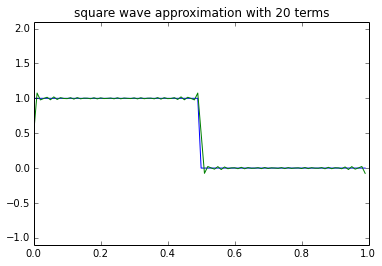A further question one might ask is: what is the link between the Fourier series analysis shown here and the discrete Fourier transform of a rectangular wave pulse?

In :
rect_fft = fft.fft(x<1/2.)
In :
sample_freq = x.size / 1.
plot(linspace(0, 1, rect_fft.size) * sample_freq, abs(rect_fft))
xlabel('frequency (Hz)')
ylabel('amplitude')
xlim(0, sample_freq / 2)
Out:
(0, 50.0)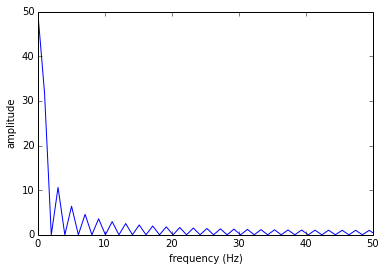As one can see in the plot above, sampling at the natural frequency of the rectangle pulse, every other harmonic component is equal to zero: this is the link with the formula above. In other words, the freqency of the rectangular pulse is 1 Hz, leading to zero components for every even frequency. We can increase the frequency resolution by zero padding our signal:

In :
zero_padded_fft = fft.fft(hstack((x<1/2., zeros(x.shape * 5))))
In :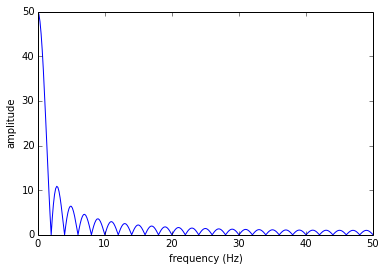Conclusion: "for a rectangle pulse of width $T$, the first zero frequency content is at $1/T * 2$"